# Mechanics, Calculus and 3D Vectors ($\mathcal{David ~ Morin}$)

$\textbf{Problem Statement}$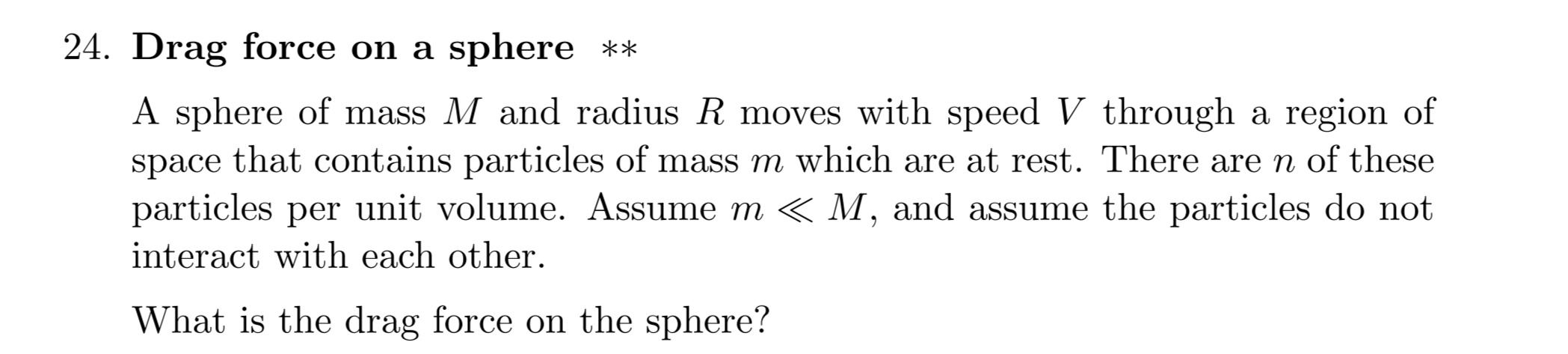$\mathbf{Attempt}$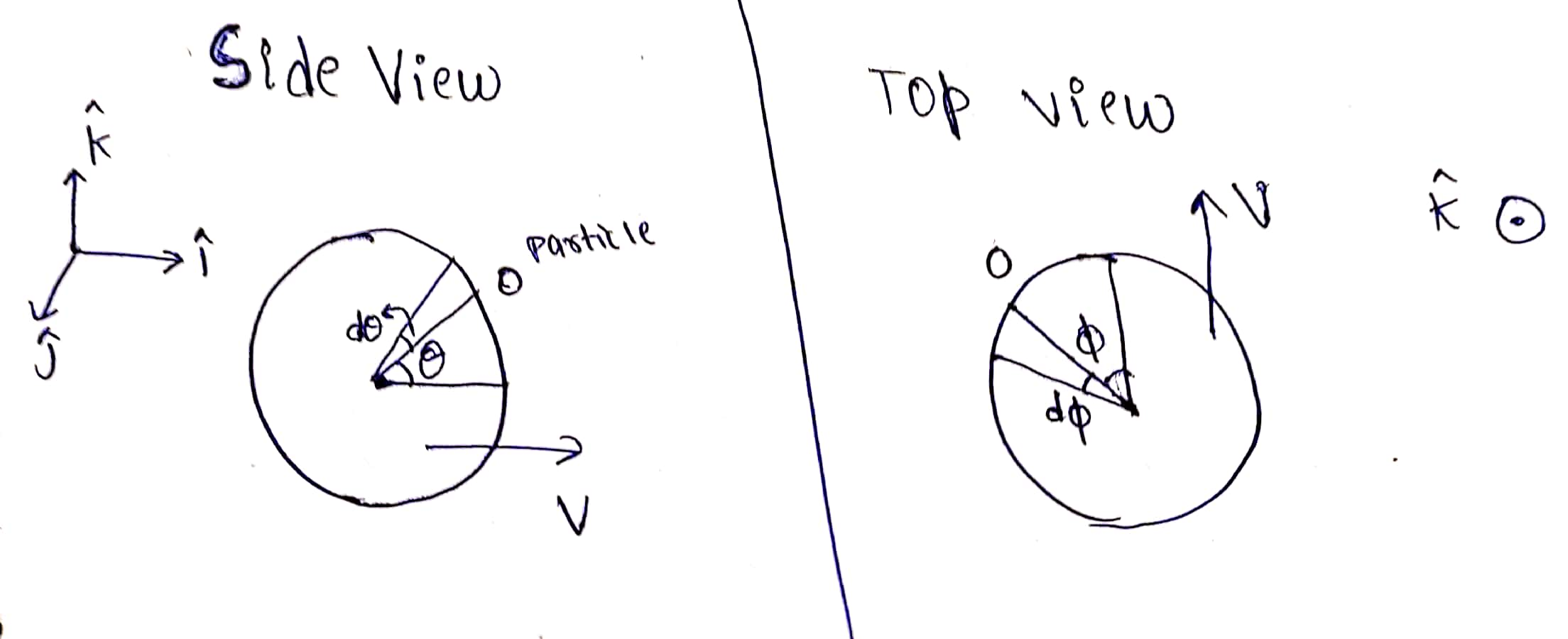Assume that the sphere is moving along the X axis.

Let the particle strike the sphere such that the radius vector of point of collision makes an angle $\theta$ with the horizontal plane (the xy plane) and its projection on xy plane make an angle $\phi$ with X axis. For the collision, the velocity of the sphere in the xy plane along the line of impact is $v \cos \phi$. We have now reduced a 3D oblique collision into a 2D one. This velocity's projection on the line of impact is $\boxed{u=v \cos \phi \cos \theta}$. Thus the collision takes place along the radius vector whose direction is $\hat{r}=\cos \phi \cos \theta \hat{i} - \sin \phi \cos \theta \hat{j} + \sin \theta \hat{k}$ ow since the sphere is large, final velocity of particle along $\hat{r}$ is $2u$, and along the X direction, the total change in momentum of particle is $\Delta P_x= mu_x=\boxed{2mv \cos^2 \phi \cos^2 \theta}$ Now the volume swept out by the region in time $\Delta t$ is $dV= vR^2 \Delta t \cos \phi \cos \theta { ~d \phi }{ ~d \theta }$ and the number of particles hitting is $n \cdot dV$, thus the total force acting on the sphere along X axis is $F= 2mv^2nR^2 \color{#3D99F6}{\underbrace{\color{#D61F06}\int_{-\pi /2}^{\pi /2} \int_{-\pi /2}^{\pi /2} \cos^3 \phi \cos^3 \theta ~d \phi ~d \theta}}_{I}}$ We can integrate over $\phi$ for a particular $\theta$ and then integrate over $\theta$.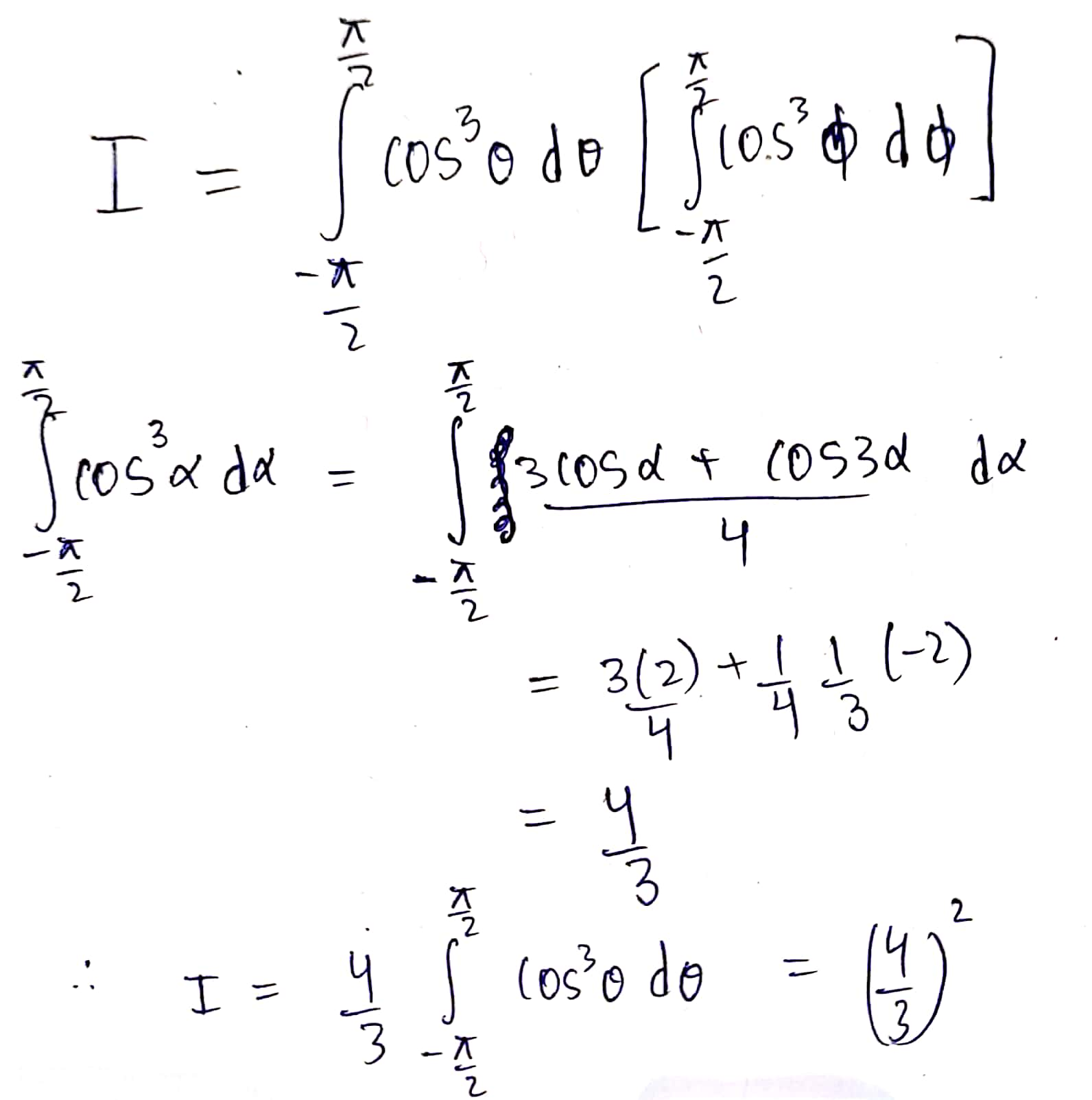So $\boxed{F=\dfrac{32}{9}mv^2nR^2}$. $\textbf{Official Solution}$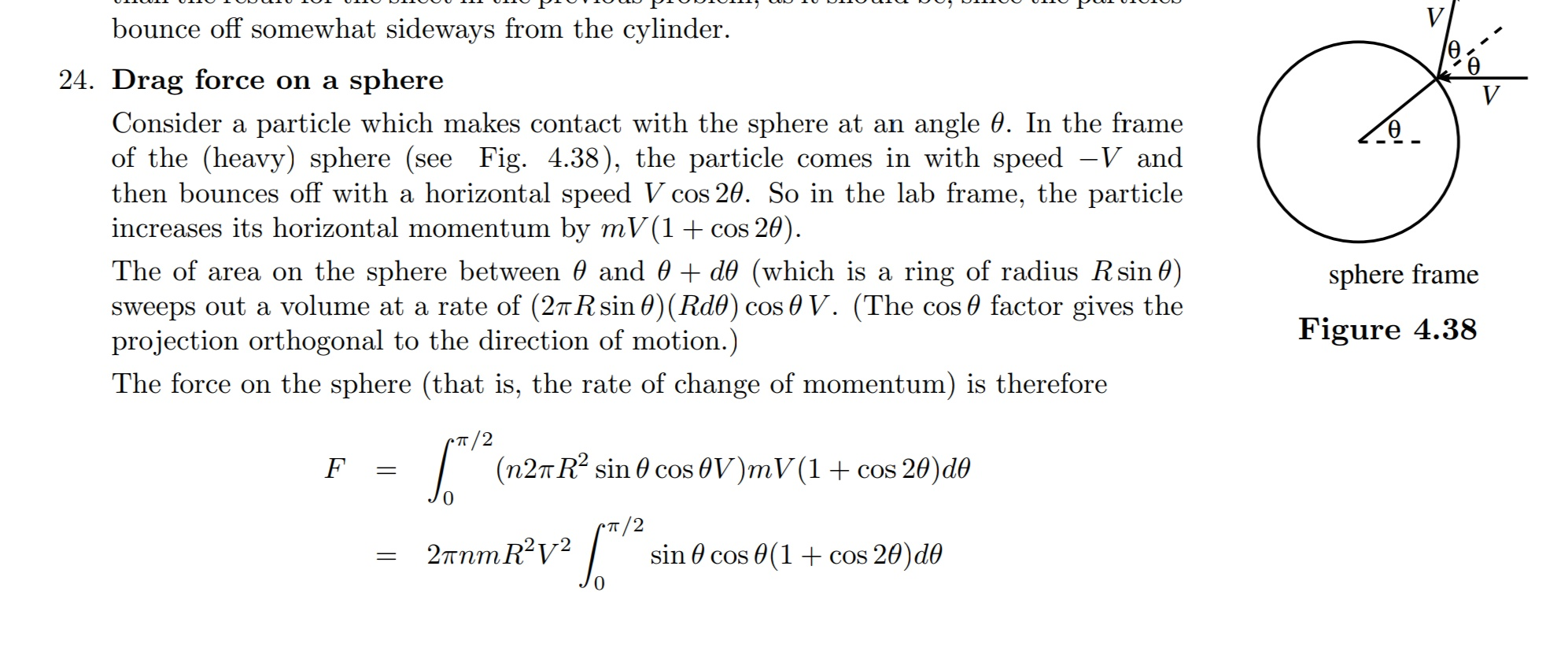I don't quite get how the theta $(\theta)$ is taken here, (from which axis or plane) and also the answer obtained is different from mine by a numerical factor ( 32/9 Vs $\pi$). Please help on this.

# Mechanics #Momentum #JEEPhysics #Calculus #Physics #DoubleIntegrals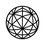Note by Harsh Poonia
1 year, 6 months ago

This discussion board is a place to discuss our Daily Challenges and the math and science related to those challenges. Explanations are more than just a solution — they should explain the steps and thinking strategies that you used to obtain the solution. Comments should further the discussion of math and science.

When posting on Brilliant:

• Use the emojis to react to an explanation, whether you're congratulating a job well done , or just really confused .
• Ask specific questions about the challenge or the steps in somebody's explanation. Well-posed questions can add a lot to the discussion, but posting "I don't understand!" doesn't help anyone.
• Try to contribute something new to the discussion, whether it is an extension, generalization or other idea related to the challenge.
• Stay on topic — we're all here to learn more about math and science, not to hear about your favorite get-rich-quick scheme or current world events.

MarkdownAppears as
*italics* or _italics_ italics
**bold** or __bold__ bold
- bulleted- list
• bulleted
• list
1. numbered2. list
1. numbered
2. list
Note: you must add a full line of space before and after lists for them to show up correctly
paragraph 1paragraph 2

paragraph 1

paragraph 2

[example link](https://brilliant.org)example link
> This is a quote
This is a quote
    # I indented these lines
# 4 spaces, and now they show
# up as a code block.

print "hello world"
# I indented these lines
# 4 spaces, and now they show
# up as a code block.

print "hello world"
MathAppears as
Remember to wrap math in $$ ... $$ or $ ... $ to ensure proper formatting.
2 \times 3 $2 \times 3$
2^{34} $2^{34}$
a_{i-1} $a_{i-1}$
\frac{2}{3} $\frac{2}{3}$
\sqrt{2} $\sqrt{2}$
\sum_{i=1}^3 $\sum_{i=1}^3$
\sin \theta $\sin \theta$
\boxed{123} $\boxed{123}$

## Comments

Sort by:

Top Newest

@Gordon Chan @Steven Chase @Mark Hennings sir please help.

- 1 year, 6 months ago

Log in to reply

Lol a callout for help for the best physicists and engineers on brilliant.org (not to mention Karan Chatrath)

- 11 months, 3 weeks ago

Log in to reply

@Md Zuhair please help.

- 1 year, 6 months ago

Log in to reply

Sorry bro... I have not checked Brilliant from 4 months now! Sorry to see this late...

- 1 year, 4 months ago

Log in to reply

Its ok. Any inputs on the problem?

- 1 year, 4 months ago

Log in to reply

×

Problem Loading...

Note Loading...

Set Loading...